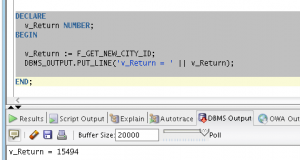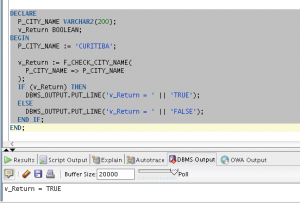# PL/SQL For Practitioners – #6 Functions

Hi folks,

user-defined function is a set of PL/SQL statements you can call by name. Functions are very similar to procedures, except that a function returns a value to the environment in which it is called.

## Syntax

```CREATE OR REPLACE
FUNCTION <name> (<paramete1_name parameter1_type, .. ,parametern_name parametern_type>) RETURN <return type>
AS
--local variables(optional)
BEGIN
END <name>;
```

## Example 1

The following example is a function responsible to return the next available city id from the table city. It is a simple algorithm, but enough to demonstrate the usage of this feature.

You can use the following script to create and populate this table. create_and_populate_tables_city_and_country

### Function

```CREATE OR REPLACE
FUNCTION F_GET_NEW_CITY_ID RETURN NUMBER AS
v_new_id number := 0;
v_is_an_used_id varchar2(1) := 'N';
BEGIN

select max(nvl(id, 0)) + 1 into v_new_id from city;

LOOP

BEGIN
select 'Y' into v_is_an_used_id from city where id = v_new_id;
EXCEPTION
WHEN NO_DATA_FOUND THEN
v_is_an_used_id := 'N';
END;

EXIT WHEN v_is_an_used_id = 'N';

v_new_id := v_new_id +1;

END LOOP;

RETURN v_new_id;

END F_GET_NEW_CITY_ID;
```

### How to test/execute in an anonymous block

No matter where you are executing or calling a function, you always must use a declared variable to receive the return from this function.

```DECLARE
v_Return NUMBER;
BEGIN

v_Return := F_GET_NEW_CITY_ID;
DBMS_OUTPUT.PUT_LINE('v_Return = ' || v_Return);

END;```

Result## Example 2

### Function

```create or replace
FUNCTION F_CHECK_CITY_NAME (p_city_name IN VARCHAR2) RETURN BOOLEAN AS
v_is_an_used_id varchar2(1) := 'N';
BEGIN

SELECT NVL('Y', 'N') INTO v_is_an_used_id FROM CITY WHERE UPPER(name) = UPPER(p_city_name);

IF v_is_an_used_id = 'Y' THEN
RETURN TRUE;
ELSE
RETURN FALSE;
END IF;

END F_CHECK_CITY_NAME;```

### How to test/execute in an anonymous block

```DECLARE
P_CITY_NAME VARCHAR2(200);
v_Return BOOLEAN;
BEGIN
P_CITY_NAME := 'CURITIBA';

v_Return := F_CHECK_CITY_NAME(
P_CITY_NAME => P_CITY_NAME
);
IF (v_Return) THEN
DBMS_OUTPUT.PUT_LINE('v_Return = ' || 'TRUE');
ELSE
DBMS_OUTPUT.PUT_LINE('v_Return = ' || 'FALSE');
END IF;
END;```

ResultThis post is part of a series about the most common structures in PL/SQL. You can check the rest of them in the link below.

PL/SQL for real world – 8 features that you have to know